Precalculus

# Chapter 5

PrecalculusChapter 5

### 5.1Angles

1.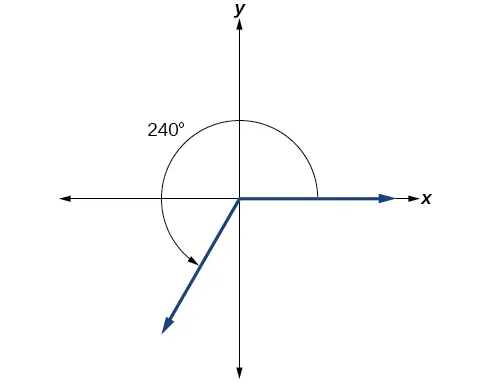2.

$3π 2 3π 2$

3.

−135°

4.

$7π 10 7π 10$

5.

$α=150° α=150°$

6.

$β=60° β=60°$

7.

$7π 6 7π 6$

8.

9.

1.88

10.

$−3π 2 −3π 2$ rad/s

11.

1655 kilometers per hour

### 5.2Unit Circle: Sine and Cosine Functions

1.

$cos(t)=− 2 2 ,sin(t)= 2 2 cos(t)=− 2 2 ,sin(t)= 2 2$

2.

$cos(π)=−1, cos(π)=−1,$ $sin(π)=0 sin(π)=0$

3.

$sin(t)=− 7 25 sin(t)=− 7 25$

4.

approximately 0.866025403

5.

$π 3 π 3$

6.
1. $cos(315°)= 2 2 , sin(315°)= – 2 2 cos(315°)= 2 2 , sin(315°)= – 2 2$
2. $cos(− π 6 )= 3 2 , sin( − π 6 )=− 1 2 cos(− π 6 )= 3 2 , sin( − π 6 )=− 1 2$
7.

$( 1 2 ,− 3 2 ) ( 1 2 ,− 3 2 )$

### 5.3The Other Trigonometric Functions

1.

$sint=− 2 2 sint=− 2 2$ , $cost= 2 2 cost= 2 2$, $tant=−1tant=−1$, $sect= 2 sect= 2$, $csct=− 2 csct=− 2$, $cott=−1 cott=−1$

2.

$sin π 3 = 3 2 sin π 3 = 3 2$, $cos π 3 = 1 2 cos π 3 = 1 2$, $tan π 3 = 3 tan π 3 = 3$, $sec π 3 =2sec π 3 =2$, $csc π 3 = 2 3 3 csc π 3 = 2 3 3$, $cot π 3 = 3 3 cot π 3 = 3 3$

3.

$sin( −7π 4 )= 2 2 ,cos( −7π 4 )= 2 2 ,tan( −7π 4 )=1, sin( −7π 4 )= 2 2 ,cos( −7π 4 )= 2 2 ,tan( −7π 4 )=1,$
$sec( −7π 4 )= 2 ,csc( −7π 4 )= 2 ,cot( −7π 4 )=1 sec( −7π 4 )= 2 ,csc( −7π 4 )= 2 ,cot( −7π 4 )=1$

4.

$− 3 − 3$

5.

$−2 −2$

6.

$sint sint$

7.

$cost=− 8 17 ,sint= 15 17 ,tant=− 15 8 cost=− 8 17 ,sint= 15 17 ,tant=− 15 8$
$csct= 17 15 ,cott=− 8 15 csct= 17 15 ,cott=− 8 15$

8.

$sint=−1,cost=0,tant=Undefined sect= Undefined,csct=−1,cott=0 sint=−1,cost=0,tant=Undefined sect= Undefined,csct=−1,cott=0$

9.

$sect= 2 ,csct= 2 ,tant=1,cott=1 sect= 2 ,csct= 2 ,tant=1,cott=1$

10.

$≈−2.414 ≈−2.414$

### 5.4Right Triangle Trigonometry

1.

$7 25 7 25$

2.

$sint= 33 65 ,cost= 56 65 ,tant= 33 56 , sect= 65 56 ,csct= 65 33 ,cott= 56 33 sint= 33 65 ,cost= 56 65 ,tant= 33 56 , sect= 65 56 ,csct= 65 33 ,cott= 56 33$

3.

$sin( π 4 )= 1 2 ,cos( π 4 )= 1 2 ,tan( π 4 )=1, sin( π 4 )= 1 2 ,cos( π 4 )= 1 2 ,tan( π 4 )=1,$
$sec( π 4 )= 2 ,csc( π 4 )= 2 ,cot( π 4 )=1 sec( π 4 )= 2 ,csc( π 4 )= 2 ,cot( π 4 )=1$

4.

2

5.

$adjacent=10; adjacent=10;$ $opposite=10 3 opposite=10 3$; missing angle is $π 6 π 6$

6.

### 5.1 Section Exercises

1.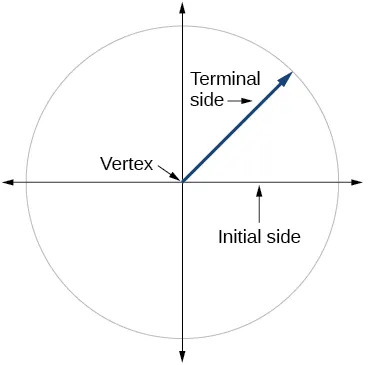3.

Whether the angle is positive or negative determines the direction. A positive angle is drawn in the counterclockwise direction, and a negative angle is drawn in the clockwise direction.

5.

Linear speed is a measurement found by calculating distance of an arc compared to time. Angular speed is a measurement found by calculating the angle of an arc compared to time.

7.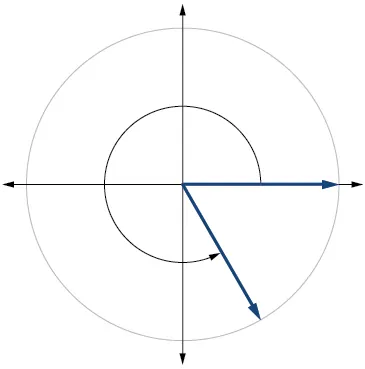9.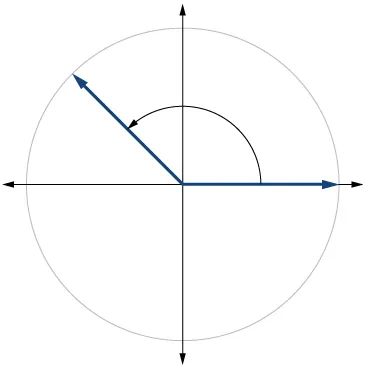11.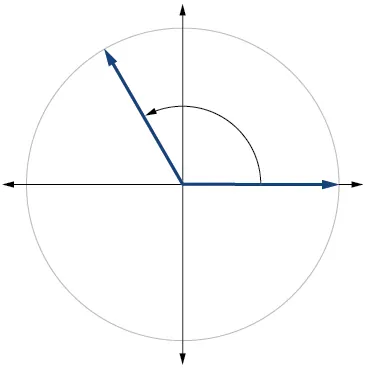13.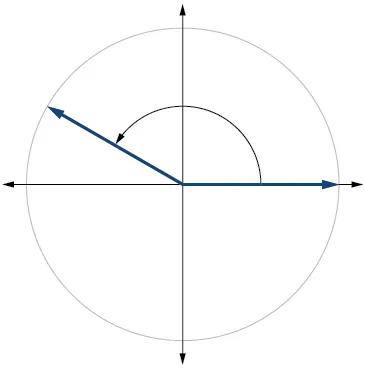15.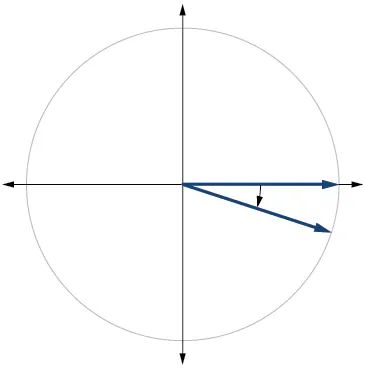17.

240°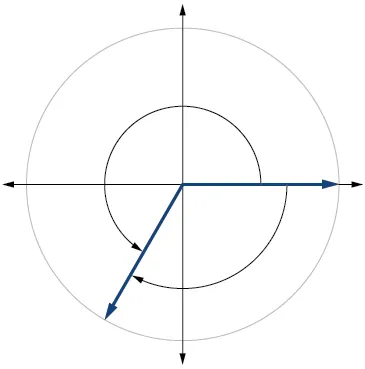19.

$4π 3 4π 3$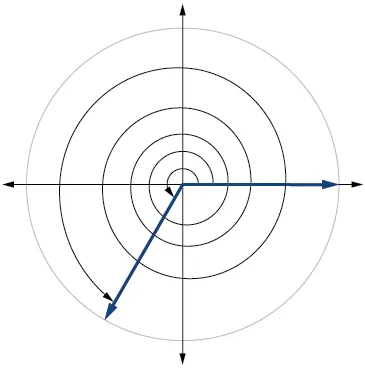21.

$2π 3 2π 3$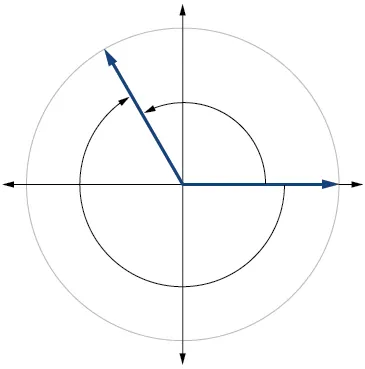23.

25.

27.

20°

29.

60°

31.

−75°

33.

$π 2 π 2$ radians

35.

$−3π −3π$ radians

37.

$π π$ radians

39.

$5π 6 5π 6$ radians

41.

$5.02π 3 ≈5.26 5.02π 3 ≈5.26$ miles

43.

$25π 9 ≈8.73 25π 9 ≈8.73$ centimeters

45.

$21π 10 ≈6.60 21π 10 ≈6.60$ meters

47.

104.7198 cm2

49.

0.7697 in2

51.

250°

53.

320°

55.

$4π 3 4π 3$

57.

$8π 9 8π 9$

59.

61.

7 in./s, 4.77 RPM, 28.65 deg/s

63.

65.

$5.76 5.76$ miles

67.

$120° 120°$

69.

794 miles per hour

71.

2,234 miles per hour

73.

11.5 inches

### 5.2 Section Exercises

1.

The unit circle is a circle of radius 1 centered at the origin.

3.

Coterminal angles are angles that share the same terminal side. A reference angle is the size of the smallest acute angle, $t, t,$ formed by the terminal side of the angle $t t$ and the horizontal axis.

5.

The sine values are equal.

7.

I

9.

IV

11.

$3 2 3 2$

13.

$1 2 1 2$

15.

$2 2 2 2$

17.

0

19.

−1

21.

$3 2 3 2$

23.

$60° 60°$

25.

$80° 80°$

27.

$45° 45°$

29.

$π 3 π 3$

31.

$π 3 π 3$

33.

$π 8 π 8$

35.

$60°, 60°,$ Quadrant IV, $sin(300°)=− 3 2 ,cos(300°)= 1 2 sin(300°)=− 3 2 ,cos(300°)= 1 2$

37.

$45°, 45°,$ Quadrant II, $sin(135°)= 2 2 , sin(135°)= 2 2 ,$ $cos(135°)=− 2 2 cos(135°)=− 2 2$

39.

$60°, 60°,$ Quadrant II, $sin(120°)= 3 2 , sin(120°)= 3 2 ,$ $cos(120°)=− 1 2 cos(120°)=− 1 2$

41.

$30°, 30°,$ Quadrant II, $sin(150°)= 1 2 , sin(150°)= 1 2 ,$ $cos(150°)=− 3 2 cos(150°)=− 3 2$

43.

$π 6 , π 6 ,$ Quadrant III, $sin( 7π 6 )=− 1 2 , sin( 7π 6 )=− 1 2 ,$ $cos( 7π 6 )=− 3 2 cos( 7π 6 )=− 3 2$

45.

$π 4 , π 4 ,$ Quadrant II, $sin( 3π 4 )= 2 2 , sin( 3π 4 )= 2 2 ,$ $cos( 4π 3 )=− 2 2 cos( 4π 3 )=− 2 2$

47.

$π 3 , π 3 ,$ Quadrant II, $sin( 2π 3 )= 3 2 , sin( 2π 3 )= 3 2 ,$ $cos( 2π 3 )=− 1 2 cos( 2π 3 )=− 1 2$

49.

$π 4 , π 4 ,$ Quadrant IV, $sin( 7π 4 )=− 2 2 , sin( 7π 4 )=− 2 2 ,$ $cos( 7π 4 )= 2 2 cos( 7π 4 )= 2 2$

51.

$77 9 77 9$

53.

$− 15 4 − 15 4$

55.

$( −10,10 3 ) ( −10,10 3 )$

57.

$( –2.778,15.757 ) ( –2.778,15.757 )$

59.

$[ –1,1 ] [ –1,1 ]$

61.

$sint= 1 2 ,cost=− 3 2 sint= 1 2 ,cost=− 3 2$

63.

$sint=− 2 2 ,cost=− 2 2 sint=− 2 2 ,cost=− 2 2$

65.

$sint= 3 2 ,cost=− 1 2 sint= 3 2 ,cost=− 1 2$

67.

$sint=− 2 2 ,cost= 2 2 sint=− 2 2 ,cost= 2 2$

69.

$sint=0,cost=−1 sint=0,cost=−1$

71.

$sint=−0.596,cost=0.803 sint=−0.596,cost=0.803$

73.

$sint= 1 2 ,cost= 3 2 sint= 1 2 ,cost= 3 2$

75.

$sint=− 1 2 ,cost= 3 2 sint=− 1 2 ,cost= 3 2$

77.

$sint=0.761,cost=−0.649 sint=0.761,cost=−0.649$

79.

$sint=1,cost=0 sint=1,cost=0$

81.

−0.1736

83.

0.9511

85.

−0.7071

87.

−0.1392

89.

−0.7660

91.

$2 4 2 4$

93.

$− 6 4 − 6 4$

95.

$2 4 2 4$

97.

$2 4 2 4$

99.

0

101.

$( 0,–1 ) ( 0,–1 )$

103.

37.5 seconds, 97.5 seconds, 157.5 seconds, 217.5 seconds, 277.5 seconds, 337.5 seconds

### 5.3 Section Exercises

1.

Yes, when the reference angle is $π 4 π 4$ and the terminal side of the angle is in quadrants I and III. Thus, at $x= π 4 , 5π 4 , x= π 4 , 5π 4 ,$ the sine and cosine values are equal.

3.

Substitute the sine of the angle in for $y y$ in the Pythagorean Theorem $x 2 + y 2 =1. x 2 + y 2 =1.$ Solve for $x x$ and take the negative solution.

5.

The outputs of tangent and cotangent will repeat every $π π$ units.

7.

$2 3 3 2 3 3$

9.

$3 3$

11.

$2 2$

13.

1

15.

2

17.

$3 3 3 3$

19.

$− 2 3 3 − 2 3 3$

21.

$3 3$

23.

$− 2 − 2$

25.

−1

27.

−2

29.

$− 3 3 − 3 3$

31.

2

33.

$3 3 3 3$

35.

−2

37.

−1

39.

If $sint=− 2 2 3 sint=− 2 2 3$, $sect=−3sect=−3$, $csct=− 3 2 4 csct=− 3 2 4$, $tant=2 2 tant=2 2$, $cott= 2 4 cott= 2 4$

41.

$sect=2 sect=2$, $csct= 2 3 3 csct= 2 3 3$, $tant= 3 tant= 3$, $cott= 3 3 cott= 3 3$

43.

$− 2 2 − 2 2$

45.

3.1

47.

1.4

49.

$sint= 2 2 ,cost= 2 2 ,tant=1,cott=1,sect= 2 ,csct= 2 sint= 2 2 ,cost= 2 2 ,tant=1,cott=1,sect= 2 ,csct= 2$

51.

$sint=− 3 2 sint=− 3 2$, $cost=− 1 2 cost=− 1 2$, $tant= 3 ,cott= 3 3 tant= 3 ,cott= 3 3$, $sect=−2sect=−2$, $csct=− 2 3 3 csct=− 2 3 3$

53.

–0.228

55.

–2.414

57.

1.414

59.

1.540

61.

1.556

63.

$sin( t )≈0.79 sin( t )≈0.79$

65.

$csct≈1.16 csct≈1.16$

67.

even

69.

even

71.

$sint cost =tant sint cost =tant$

73.

13.77 hours, period: $1000π 1000π$

75.

7.73 inches

### 5.4 Section Exercises

1.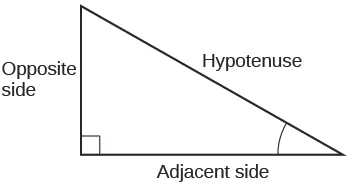3.

The tangent of an angle is the ratio of the opposite side to the adjacent side.

5.

For example, the sine of an angle is equal to the cosine of its complement; the cosine of an angle is equal to the sine of its complement.

7.

$π 6 π 6$

9.

$π 4 π 4$

11.

$b= 20 3 3 ,c= 40 3 3 b= 20 3 3 ,c= 40 3 3$

13.

$a=10,000,c=10,000.5 a=10,000,c=10,000.5$

15.

$b= 5 3 3 ,c= 10 3 3 b= 5 3 3 ,c= 10 3 3$

17.

$5 29 29 5 29 29$

19.

$5 2 5 2$

21.

$29 2 29 2$

23.

$5 41 41 5 41 41$

25.

$5 4 5 4$

27.

$41 4 41 4$

29.

$c=14,b=7 3 c=14,b=7 3$

31.

$a=15,b=15 a=15,b=15$

33.

$b=9.9970,c=12.2041 b=9.9970,c=12.2041$

35.

$a=2.0838,b=11.8177 a=2.0838,b=11.8177$

37.

$a=55.9808,c=57.9555 a=55.9808,c=57.9555$

39.

$a=46.6790,b=17.9184 a=46.6790,b=17.9184$

41.

$a=16.4662,c=16.8341 a=16.4662,c=16.8341$

43.

188.3159

45.

200.6737

47.

498.3471 ft

49.

1060.09 ft

51.

27.372 ft

53.

22.6506 ft

55.

368.7633 ft

### Review Exercises

1.

$45° 45°$

3.

$− 7π 6 − 7π 6$

5.

10.385 meters

7.

$60° 60°$

9.

$2π 11 2π 11$

11.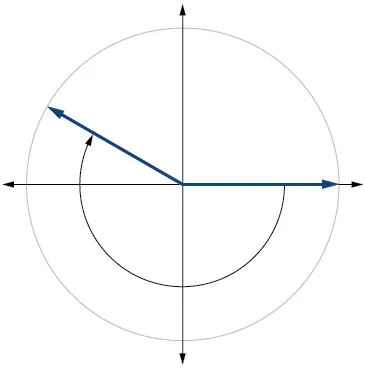13.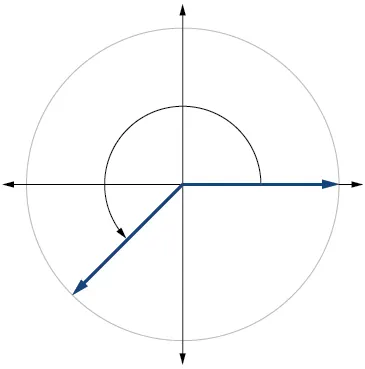15.

1036.73 miles per hour

17.

$3 2 3 2$

19.

–1

21.

$π 4 π 4$

23.

$− 2 2 − 2 2$

25.

$[ –1,1 ] [ –1,1 ]$

27.

1

29.

$2 2$

31.

$2 2$

33.

0.6

35.

$2 2 2 2$ or $− 2 2 − 2 2$

37.

sine, cosecant, tangent, cotangent

39.

$3 3 3 3$

41.

0

43.

$b=8,c=10 b=8,c=10$

45.

$11 157 157 11 157 157$

47.

$a=4,b=4 a=4,b=4$

49.

14.0954 ft

### Practice Test

1.

$150° 150°$

3.

6.283 centimeters

5.

$15° 15°$

7.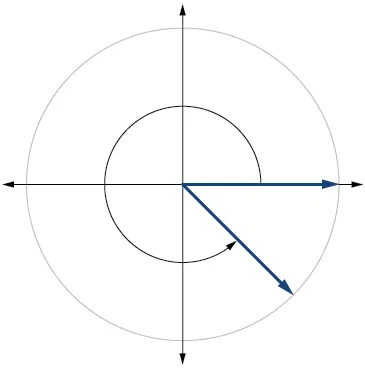9.

3.351 feet per second, $2π 75 2π 75$ radians per second

11.

$− 3 2 − 3 2$

13.

$[ –1,1 ] [ –1,1 ]$

15.

$3 3$

17.

$3 3 3 3$

19.

$3 2 3 2$

21.

$π 3 π 3$

23.

$a= 9 2 ,b= 9 3 2 a= 9 2 ,b= 9 3 2$

Order a print copy

As an Amazon Associate we earn from qualifying purchases.